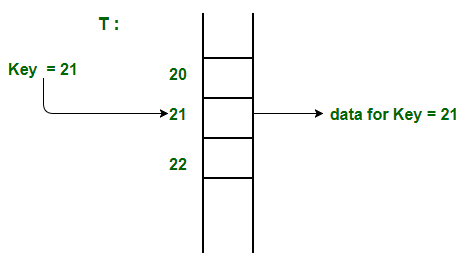# Index Mapping (or Trivial Hashing) with negatives allowed

• Difficulty Level : Easy
• Last Updated : 21 Jul, 2022

Given a limited range array contains both positive and non-positive numbers, i.e., elements are in the range from -MAX to +MAX. Our task is to search if some number is present in the array or not in O(1) time.
Since range is limited, we can use index mapping (or trivial hashing). We use values as the index in a big array. Therefore we can search and insert elements in O(1) time.How to handle negative numbers?
The idea is to use a 2D array of size hash[MAX+1]

Algorithm:

Assign all the values of the hash matrix as 0.

Traverse the given array:

•     If the element ele is non negative assign
• hash[ele] as 1.
•     Else take the absolute value of ele and
•  assign hash[ele] as 1.

To search any element x in the array.

• If X is non-negative check if hash[X] is 1 or not. If hash[X] is one then the number is present else not present.
• If X is negative take absolute value of X and then check if hash[X] is 1 or not. If hash[X] is one then the number is present

Below is the implementation of the above idea.

## C++

 `// CPP program to implement direct index mapping``// with negative values allowed.``#include ``using` `namespace` `std;``#define MAX 1000` `// Since array is global, it is initialized as 0.``bool` `has[MAX + 1];` `// searching if X is Present in the given array``// or not.``bool` `search(``int` `X)``{``    ``if` `(X >= 0) {``        ``if` `(has[X] == 1)``            ``return` `true``;``        ``else``            ``return` `false``;``    ``}` `    ``// if X is negative take the absolute``    ``// value of X.``    ``X = ``abs``(X);``    ``if` `(has[X] == 1)``        ``return` `true``;` `    ``return` `false``;``}` `void` `insert(``int` `a[], ``int` `n)``{``    ``for` `(``int` `i = 0; i < n; i++) {``        ``if` `(a[i] >= 0)``            ``has[a[i]] = 1;``       ``else``            ``has[``abs``(a[i])] = 1;``    ``}``}` `// Driver code``int` `main()``{``    ``int` `a[] = { -1, 9, -5, -8, -5, -2 };``    ``int` `n = ``sizeof``(a)/``sizeof``(a);``    ``insert(a, n);``    ``int` `X = -5;``    ``if` `(search(X) == ``true``)``       ``cout << ``"Present"``;``    ``else``       ``cout << ``"Not Present"``;``    ``return` `0;``}`

## Java

 `// Java program to implement direct index``// mapping with negative values allowed.``class` `GFG``{` `final` `static` `int` `MAX = ``1000``;` `// Since array is global, it``// is initialized as 0.``static` `boolean``[][] has = ``new` `boolean``[MAX + ``1``][``2``];` `// searching if X is Present in``// the given array or not.``static` `boolean` `search(``int` `X)``{``    ``if` `(X >= ``0``)``    ``{``        ``if` `(has[X][``0``] == ``true``)``        ``{``            ``return` `true``;``        ``}``        ``else``        ``{``            ``return` `false``;``        ``}``    ``}` `    ``// if X is negative take the``    ``// absolute value of X.``    ``X = Math.abs(X);``    ``if` `(has[X][``1``] == ``true``)``    ``{``        ``return` `true``;``    ``}` `    ``return` `false``;``}` `static` `void` `insert(``int` `a[], ``int` `n)``{``    ``for` `(``int` `i = ``0``; i < n; i++)``    ``{``        ``if` `(a[i] >= ``0``)``        ``{``            ``has[a[i]][``0``] = ``true``;``        ``}``        ``else``        ``{``            ``int` `abs_i = Math.Abs(a[i]);``            ``has[abs_i][``1``] = ``true``;``        ``}``    ``}``}` `// Driver code``public` `static` `void` `main(String args[])``{``    ``int` `a[] = {-``1``, ``9``, -``5``, -``8``, -``5``, -``2``};``    ``int` `n = a.length;``    ``insert(a, n);``    ``int` `X = -``5``;``    ``if` `(search(X) == ``true``)``    ``{``        ``System.out.println(``"Present"``);``    ``}``    ``else``    ``{``        ``System.out.println(``"Not Present"``);``    ``}``}``}` `// This code is contributed``// by 29AjayKumar`

## Python3

 `# Python3 program to implement direct index``# mapping with negative values allowed.` `# Searching if X is Present in the``# given array or not.``def` `search(X):` `    ``if` `X >``=` `0``:``        ``return` `has[X][``0``] ``=``=` `1` `    ``# if X is negative take the absolute``    ``# value of X.``    ``X ``=` `abs``(X)``    ``return` `has[X][``1``] ``=``=` `1` `def` `insert(a, n):` `    ``for` `i ``in` `range``(``0``, n):``        ``if` `a[i] >``=` `0``:``            ``has[a[i]][``0``] ``=` `1``        ``else``:``            ``has[``abs``(a[i])][``1``] ``=` `1` `# Driver code``if` `__name__ ``=``=` `"__main__"``:` `    ``a ``=` `[``-``1``, ``9``, ``-``5``, ``-``8``, ``-``5``, ``-``2``]``    ``n ``=` `len``(a)` `    ``MAX` `=` `1000``    ` `    ``# Since array is global, it is``    ``# initialized as 0.``    ``has ``=` `[[``0` `for` `i ``in` `range``(``2``)]``              ``for` `j ``in` `range``(``MAX` `+` `1``)]``    ``insert(a, n)` `    ``X ``=` `-``5``    ``if` `search(X) ``=``=` `True``:``        ``print``(``"Present"``)``    ``else``:``        ``print``(``"Not Present"``)` `# This code is contributed by Rituraj Jain`

## C#

 `// C# program to implement direct index``// mapping with negative values allowed.``using` `System;` `class` `GFG``{` `static` `int` `MAX = 1000;` `// Since array is global, it``// is initialized as 0.``static` `bool``[,] has = ``new` `bool``[MAX + 1, 2];` `// searching if X is Present in``// the given array or not.``static` `bool` `search(``int` `X)``{``    ``if` `(X >= 0)``    ``{``        ``if` `(has[X, 0] == ``true``)``        ``{``            ``return` `true``;``        ``}``        ``else``        ``{``            ``return` `false``;``        ``}``    ``}` `    ``// if X is negative take the``    ``// absolute value of X.``    ``X = Math.Abs(X);``    ``if` `(has[X, 1] == ``true``)``    ``{``        ``return` `true``;``    ``}` `    ``return` `false``;``}` `static` `void` `insert(``int``[] a, ``int` `n)``{``    ``for` `(``int` `i = 0; i < n; i++)``    ``{``        ``if` `(a[i] >= 0)``        ``{``            ``has[a[i], 0] = ``true``;``        ``}``        ``else``        ``{   ``            ``int` `abs_i = Math.Abs(a[i]);``            ``has[abs_i, 1] = ``true``;``        ``}``    ``}``}` `// Driver code``public` `static` `void` `Main()``{``    ``int``[] a = {-1, 9, -5, -8, -5, -2};``    ``int` `n = a.Length;``    ``insert(a, n);``    ``int` `X = -5;``    ``if` `(search(X) == ``true``)``    ``{``        ``Console.WriteLine(``"Present"``);``    ``}``    ``else``    ``{``        ``Console.WriteLine(``"Not Present"``);``    ``}``}``}` `// This code is contributed``// by Akanksha Rai`

## Javascript

 ``

Output

`Present`

This article is contributed by ShivamKD. If you like GeeksforGeeks and would like to contribute, you can also write an article using write.geeksforgeeks.org or mail your article to review-team@geeksforgeeks.org. See your article appearing on the GeeksforGeeks main page and help other Geeks.

My Personal Notes arrow_drop_up